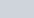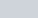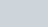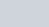Write the first five terms of each of the following sequences whose nth terms are:
Question:

Write the first five terms of each of the following sequences whose nth terms are:

(a) $a_{n}=3 n+2$

(b) $a_{n}=\frac{n-3}{3}$

(c) $a_{n}=3^{n}$

(d) $a_{n}=\frac{3 n-2}{5}$

(e) $a_{n}=(-1)^{n} 2^{n}$

(f) $a_{n}=\frac{n(n-2)}{2}$

(g) an $=n^{2}-n+1$

(h) $a_{n}=2 n^{2}-3 n+1$

(i) $a_{n}=\frac{2 n-3}{6}$

Solution:

Here, we are given the nth term for various sequences. We need to find the first five terms of the sequence.

(i) $a_{n}=3 n+2$

Here, the nth term is given by the above expression. So, to find the first term we use, we get,

$a_{1}=3(1)+2$

$=3+2$

$=5$

Similarly, we find the other four terms,

Second term (),

$a_{2}=3(2)+2$

$=6+2$

$=8$

Third term $(n=3)$,

$a_{3}=3(3)+2$

$=9+2$

$=11$

Fourth term $(n=4)$,

$a_{4}=3(4)+2$

$=12+2$

$=14$

Fifth term $(n=5)$,

$a_{5}=3(5)+2$

$=15+2$

$=17$

Therefore, the first five terms for the given sequence are $a_{1}=5, a_{2}=8, a_{3}=11, a_{4}=14, a_{5}=17$.

(ii) $a_{n}=\frac{n-2}{3}$

Here, the nth term is given by the above expression. So, to find the first term we use,, we get,

$a_{1}=\frac{(1)-2}{3}$

$=\frac{-1}{3}$

Similarly, we find the other four terms,

Second term (),

$a_{2}=\frac{(2)-2}{3}$

$=\frac{0}{3}$

$=0$

Third term,

$a_{3}=\frac{(3)-2}{3}$

$=\frac{1}{3}$

Fourth term,

$a_{4}=\frac{(4)-2}{3}$

$=\frac{2}{3}$

Fifth term $(n=5)$,

$a_{5}=\frac{(5)-2}{3}$

$=\frac{3}{3}$

$=1$

Therefore, the first five terms for the given sequence are $a_{1}=\frac{-1}{3}, a_{2}=0, a_{3}=\frac{1}{3}, a_{4}=\frac{2}{3}, a_{5}=1$.

(iii) $a_{n}=3^{n}$

Here, the nth term is given by the above expression. So, to find the first term we use, we get,

$a_{1}=3^{(1)}$

$=3$

Similarly, we find the other four terms,

Second term (),

$a_{2}=3^{(2)}$

$=(3)(3)$

$=9$

Third term $(n=3)$,

$a_{3}=3^{(3)}$

$=(3)(3)(3)$

$=27$

Fourth term,

$a_{4}=3^{(4)}$

$=(3)(3)(3)(3)$

$=81$

Fifth term $(n=5)$,

$a_{5}=3^{(5)}$

$=(3)(3)(3)(3)(3)$

$=243$

Therefore, the first five terms for the given sequence are $a_{1}=3, a_{2}=9, a_{3}=27, a_{4}=81, a_{5}=243$.

(iv) $a_{n}=\frac{3 n-2}{5}$

Here, the nth term is given by the above expression. So, to find the first term we use,, we get,

$a_{1}=\frac{3(1)-2}{5}$

$=\frac{1}{5}$

Similarly, we find the other four terms,

Second term $(n=2)$,

$a_{2}=\frac{3(2)-2}{5}$

$=\frac{6-2}{5}$

$=\frac{4}{5}$

Third term,

$a_{3}=\frac{3(3)-2}{5}$

$=\frac{9-2}{5}$

$=\frac{7}{5}$

Fourth term $(n=4)$,

$a_{4}=\frac{3(4)-2}{5}$

$=\frac{12-2}{5}$

$=\frac{10}{5}$

$=2$

Fifth term $(n=5)$,

$a_{5}=\frac{3(5)-2}{5}$

$=\frac{15-2}{5}$

$=\frac{13}{5}$

Therefore, the first five terms for the given sequence are $a_{1}=\frac{1}{5}, a_{2}=\frac{4}{5}, a_{3}=\frac{7}{5}, a_{4}=2, a_{5}=\frac{13}{5}$.

(v) $a_{n}=(-1)^{n} \cdot 2^{n}$

Here, the nth term is given by the above expression. So, to find the first term we use, we get,

$a_{1}=(-1)^{1} \cdot 2^{1}$

$=(-1) \cdot 2$

$=-2$

Similarly, we find the other four terms,

Second term $(n=2)$

$a_{2}=(-1)^{2} \cdot 2^{2}$

$=1.4$

$=4$

Third term $(n=3)$,

$a_{3}=(-1)^{3} \cdot 2^{3}$

$=(-1) .8$

$=-8$

Fourth term $(n=4)$,

$a_{4}=(-1)^{4} \cdot 2^{4}$

$=1.16$

$=16$

Fifth term $(n=5)$,

$a_{5}=(-1)^{5} \cdot 2^{5}$

$=(-1) .32$

$=-32$

Therefore, the first five terms of the given A.P are $a_{1}=-2, a_{2}=4, a_{3}=-8, a_{4}=16, a_{5}=-32$.

(vi) $a_{s}=\frac{n(n-2)}{2}$

Here, the nth term is given by the above expression. So, to find the first term we use, we get,

$a_{1}=\frac{1(1-2)}{2}$

$=\frac{-1}{2}$

Similarly, we find the other four terms,

Second term (),

$a_{2}=\frac{2(2-2)}{2}$

$=\frac{2(0)}{2}$

$=0$

Third term $(n=3)$,

$a_{3}=\frac{3(3-2)}{2}$

$=\frac{3(1)}{2}$

$=\frac{3}{2}$

Fourth term $(n=4)$,

$a_{4}=\frac{4(4-2)}{2}$

$=\frac{4(2)}{2}$

$=\frac{8}{2}$

$=4$

Fifth term $(n=5)$,

$a_{5}=\frac{5(5-2)}{2}$

$=\frac{5(3)}{2}$

$=\frac{15}{2}$

Therefore, the first five terms for the given sequence are $a_{1}=\frac{-1}{2}, a_{2}=0, a_{3}=\frac{3}{2}, a_{4}=4, a_{5}=\frac{15}{2}$.

(vii) $a_{n}=n^{2}-n+1$

Here, the nth term is given by the above expression. So, to find the first term we use, we get,

$a_{1}=(1)^{2}-(1)+1$

$=1-1+1$

$=1$

Similarly, we find the other four terms,

Second term (),

$a_{2}=(2)^{2}-(2)+1$

$=4-2+1$

$=3$

Third term $(n=3)$,

$a_{3}=(3)^{2}-(3)+1$

$=9-3+1$

$=13$

Fifth term $(n=5)$,

$a_{5}=(5)^{2}-(5)+1$

$=25-5+1$

$=21$

Therefore, the first five terms for the given sequence are $a_{1}=1, a_{2}=3, a_{3}=7, a_{4}=13, a_{5}=21$.

(viii) $a_{n}=2 n^{2}-3 n+1$

Here, the $n^{\text {th }}$ term is given by the above expression. So, to find the first term we use $n=1$, we get,

$a_{1}=2(1)^{2}-3(1)+1$

$=2(1)-3+1$

$=2-3+1$

$=0$

Similarly, we find the other four terms,

Second term (),

$a_{2}=2(2)^{2}-3(2)+1$

$=2(4)-6+1$

$=8-6+1$

$=3$

Third term $(n=3)$,

$a_{3}=2(3)^{2}-3(3)+1$

$=2(9)-9+1$

$=18-9+1$

$=10$

Fourth term $(n=4)$,

$=2(16)-12+1$

$=32-12+1$

$=21$

Fifth term $(n=5)$,

$a_{5}=2(5)^{2}-3(5)+1$

$=2(25)-15+1$

$=50-15+1$

$=36$

Therefore, the first five terms for the given sequence are $a_{1}=0, a_{2}=3, a_{3}=10, a_{4}=21, a_{5}=36$.

(ix) $a_{e}=\frac{2 n-3}{6}$

Here, the nth term is given by the above expression. So, to find the first term we use, we get,

$a_{1}=\frac{2(1)-3}{6}$

$=\frac{2-3}{6}$

$=\frac{-1}{6}$

Similarly, we find the other four terms,

Second term (),

$a_{2}=\frac{2(2)-3}{6}$

$=\frac{4-3}{6}$

$=\frac{1}{6}$

Third term $(n=3)$,

$a_{3}=\frac{2(3)-3}{6}$

$=\frac{6-3}{6}$

$=\frac{3}{6}$

$=\frac{1}{2}$

Fourth term $(n=4)$,

$a_{4}=\frac{2(4)-3}{6}$

$=\frac{8-3}{6}$

$=\frac{5}{6}$

Fifth term $(n=5)$,

$a_{5}=\frac{2(5)-3}{6}$

$=\frac{10-5}{6}$

$=\frac{7}{6}$

Therefore, the first five terms of the given A.P are

$a_{1}=\frac{-1}{6}, a_{2}=\frac{1}{6}, a_{3}=\frac{1}{2}, a_{4}=\frac{5}{6}, a_{5}=\frac{7}{6}$